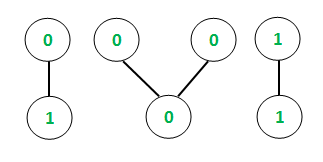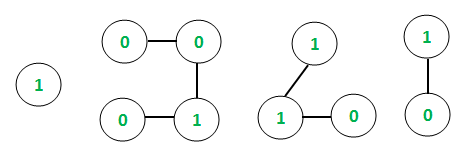Open in App
Not now

# Octal equivalents of connected components in Binary valued graph

• Last Updated : 18 Nov, 2020

Given a binary valued undirected graph with V vertices and E edges, the task is to find the octal equivalents of all the connected components of the graph. A binary valued graph can be considered as having only binary numbers (0 or 1) as the vertex values.

Examples:

Input: E = 4, V = 7Output:
Chain = 0 1      Octal equivalent = 1
Chain = 0 0 0   Octal equivalent = 0
Chain = 1 1      Octal equivalent = 3
Explanation:
In case of the first connected component, the binary chain is [0, 1]
Hence, the binary string = “01” and binary number = 01
Therefore, the octal equivalent is 1

Input: E = 6, V = 10Output:
Chain = 1      Octal equivalent = 1
Chain = 0 0 1 0      Octal equivalent = 2
Chain = 1 1 0      Octal equivalent = 6
Chain = 1 0      Octal equivalent = 2

Approach: The idea is to use Depth First Search Traversal to keep track of the connected components in the undirected graph as explained in this article. For each connected component, the binary string is displayed and the equivalent octal value is calculated from the binary value (as explained in this article) and printed.

Below is the implementation of the above approach:

## C++

 `// C++ implementation to find``// octal equivalents of``// all connected components` `#include ` `using` `namespace` `std;` `// Function to traverse the undirected``// graph using the Depth first traversal``void` `depthFirst(``int` `v, vector<``int``> graph[],``                ``vector<``bool``>& visited,``                ``vector<``int``>& storeChain)``{``    ``// Marking the visited``    ``// vertex as true``    ``visited[v] = ``true``;` `    ``// Store the connected chain``    ``storeChain.push_back(v);` `    ``for` `(``auto` `i : graph[v]) {``        ``if` `(visited[i] == ``false``) {` `            ``// Recursive call to``            ``// the DFS algorithm``            ``depthFirst(i, graph,``                       ``visited, storeChain);``        ``}``    ``}``}` `// Function to create map between binary``// number and its equivalent octal value``void` `createMap(unordered_map* um)``{``    ``(*um)[``"000"``] = ``'0'``;``    ``(*um)[``"001"``] = ``'1'``;``    ``(*um)[``"010"``] = ``'2'``;``    ``(*um)[``"011"``] = ``'3'``;``    ``(*um)[``"100"``] = ``'4'``;``    ``(*um)[``"101"``] = ``'5'``;``    ``(*um)[``"110"``] = ``'6'``;``    ``(*um)[``"111"``] = ``'7'``;``}` `// Function to return octal``// equivalent of each connected``// component``string Octal(string bin)``{``    ``int` `l = bin.size();``    ``int` `t = bin.find_first_of(``'.'``);` `    ``// length of string before '.'``    ``int` `len_left = t != -1 ? t : l;` `    ``// add min 0's in the beginning to make``    ``// left substring length divisible by 3``    ``for` `(``int` `i = 1;``         ``i <= (3 - len_left % 3) % 3;``         ``i++)``        ``bin = ``'0'` `+ bin;` `    ``// if decimal point exists``    ``if` `(t != -1) {``        ``// length of string after '.'``        ``int` `len_right = l - len_left - 1;` `        ``// add min 0's in the end to make right``        ``// substring length divisible by 3``        ``for` `(``int` `i = 1;``             ``i <= (3 - len_right % 3) % 3;``             ``i++)``            ``bin = bin + ``'0'``;``    ``}` `    ``// create map between binary and its``    ``// equivalent octal code``    ``unordered_map bin_oct_map;``    ``createMap(&bin_oct_map);` `    ``int` `i = 0;``    ``string octal = ``""``;` `    ``while` `(1) {` `        ``// one by one extract from left,``        ``// substring of size 3 and``        ``// add its octal code``        ``octal += bin_oct_map[bin.substr(i, 3)];``        ``i += 3;``        ``if` `(i == bin.size())``            ``break``;` `        ``// if '.' is encountered``        ``// add it to result``        ``if` `(bin.at(i) == ``'.'``) {``            ``octal += ``'.'``;``            ``i++;``        ``}``    ``}` `    ``// required octal number``    ``return` `octal;``}` `// Function to find the octal equivalents``// of all connected components``void` `octalValue(``    ``vector<``int``> graph[], ``int` `vertices,``    ``vector<``int``> values)``{``    ``// Initializing boolean array``    ``// to mark visited vertices``    ``vector<``bool``> visited(1001, ``false``);` `    ``// Following loop invokes DFS algorithm``    ``for` `(``int` `i = 1; i <= vertices; i++) {``        ``if` `(visited[i] == ``false``) {` `            ``// Variable to hold``            ``// temporary length``            ``int` `sizeChain;` `            ``// Container to store each chain``            ``vector<``int``> storeChain;` `            ``// DFS algorithm``            ``depthFirst(i, graph,``                       ``visited, storeChain);` `            ``// Variable to hold each chain size``            ``sizeChain = storeChain.size();` `            ``// Container to store values``            ``// of vertices of individual chains``            ``int` `chainValues[sizeChain + 1];` `            ``// Storing the values of each chain``            ``for` `(``int` `i = 0; i < sizeChain; i++) {` `                ``int` `temp``                    ``= values[storeChain[i] - 1];``                ``chainValues[i] = temp;``            ``}` `            ``// Printing binary chain``            ``cout << ``"Chain = "``;``            ``for` `(``int` `i = 0; i < sizeChain; i++) {``                ``cout << chainValues[i] << ``" "``;``            ``}``            ``cout << ``"\t"``;` `            ``// Converting the array with vertex``            ``// values to a binary string``            ``// using string stream``            ``stringstream ss;``            ``ss << chainValues;``            ``string s = ss.str();` `            ``for` `(``int` `i = 1; i < sizeChain; i++) {``                ``stringstream ss1;``                ``ss1 << chainValues[i];``                ``string s1 = ss1.str();``                ``s.append(s1);``            ``}` `            ``// Printing the octal values``            ``cout << ``"Octal equivalent = "``;``            ``cout << Octal(s) << endl;``        ``}``    ``}``}` `// Driver code to test above function``int` `main()``{``    ``// Initializing graph in the``    ``// form of adjacency list``    ``vector<``int``> graph;` `    ``// Defining the number``    ``// of edges and vertices``    ``int` `E, V;``    ``E = 4;``    ``V = 7;` `    ``// Assigning the values for each``    ``// vertex of the undirected graph``    ``vector<``int``> values;``    ``values.push_back(0);``    ``values.push_back(1);``    ``values.push_back(0);``    ``values.push_back(0);``    ``values.push_back(0);``    ``values.push_back(1);``    ``values.push_back(1);` `    ``// Constructing the undirected graph``    ``graph.push_back(2);``    ``graph.push_back(1);``    ``graph.push_back(4);``    ``graph.push_back(3);``    ``graph.push_back(5);``    ``graph.push_back(4);``    ``graph.push_back(7);``    ``graph.push_back(6);` `    ``octalValue(graph, V, values);``    ``return` `0;``}`

## Java

 `// Java implementation to find``// octal equivalents of all``// connected components``import` `java.io.*;``import` `java.util.*;` `class` `GFG{` `// Function to traverse the undirected``// graph using the Depth first traversal``static` `void` `depthFirst(``int` `v,``                       ``List> graph,``                       ``boolean``[] visited,``                       ``List storeChain)``{``    ` `    ``// Marking the visited``    ``// vertex as true``    ``visited[v] = ``true``;` `    ``// Store the connected chain``    ``storeChain.add(v);` `    ``for``(``int` `i : graph.get(v))``    ``{``        ``if` `(visited[i] == ``false``)``        ``{``            ` `            ``// Recursive call to``            ``// the DFS algorithm``            ``depthFirst(i, graph, visited,``                       ``storeChain);``        ``}``    ``}``}` `// Function to create map between binary``// number and its equivalent hexadecimal``static` `void` `createMap(Map um)``{``    ``um.put(``"000"``, ``'0'``);``    ``um.put(``"001"``, ``'1'``);``    ``um.put(``"010"``, ``'2'``);``    ``um.put(``"011"``, ``'3'``);``    ``um.put(``"100"``, ``'4'``);``    ``um.put(``"101"``, ``'5'``);``    ``um.put(``"110"``, ``'6'``);``    ``um.put(``"111"``, ``'7'``);``}` `// Function to return octal``// equivalent of each connected``// component``static` `String octal(String bin)``{``    ``int` `l = bin.length();``    ``int` `t = bin.indexOf(``'.'``);` `    ``// Length of string before '.'``    ``int` `len_left = t != -``1` `? t : l;` `    ``// Add min 0's in the beginning to make``    ``// left substring length divisible by 3``    ``for``(``int` `i = ``1``;``            ``i <= (``3` `- len_left % ``3``) % ``3``;``            ``i++)``        ``bin = ``'0'` `+ bin;` `    ``// If decimal point exists``    ``if` `(t != -``1``)``    ``{``        ` `        ``// Length of string after '.'``        ``int` `len_right = l - len_left - ``1``;` `        ``// Add min 0's in the end to make right``        ``// substring length divisible by 3``        ``for``(``int` `i = ``1``;``                ``i <= (``3` `- len_right % ``3``) % ``3``;``                ``i++)``            ``bin = bin + ``'0'``;``    ``}` `    ``// Create map between binary and its``    ``// equivalent octal code``    ``Map bin_oct_map = ``new` `HashMap();``    ``createMap(bin_oct_map);` `    ``int` `i = ``0``;``    ``String octal = ``""``;` `    ``while` `(``true``)``    ``{``        ` `        ``// One by one extract from left,``        ``// substring of size 3 and``        ``// add its octal code``        ``octal += bin_oct_map.get(``            ``bin.substring(i, i + ``3``));``            ` `        ``i += ``3``;``        ``if` `(i == bin.length())``            ``break``;` `        ``// If '.' is encountered``        ``// add it to result``        ``if` `(bin.charAt(i) == ``'.'``)``        ``{``            ``octal += ``'.'``;``            ``i++;``        ``}``    ``}` `    ``// Required octal number``    ``return` `octal;``}` `// Function to find the octal equivalents``// of all connected components``static` `void` `octalValue(List> graph,``                       ``int` `vertices,``                       ``List values)``{``    ` `    ``// Initializing boolean array``    ``// to mark visited vertices``    ``boolean``[] visited = ``new` `boolean``[``1001``];` `    ``// Following loop invokes DFS algorithm``    ``for``(``int` `i = ``1``; i <= vertices; i++)``    ``{``        ``if` `(visited[i] == ``false``)``        ``{``            ` `            ``// Variable to hold``            ``// temporary length``            ``int` `sizeChain;` `            ``// Container to store each chain``            ``List storeChain = ``new` `ArrayList();` `            ``// DFS algorithm``            ``depthFirst(i, graph, visited, storeChain);` `            ``// Variable to hold each chain size``            ``sizeChain = storeChain.size();` `            ``// Container to store values``            ``// of vertices of individual chains``            ``int``[] chainValues = ``new` `int``[sizeChain + ``1``];` `            ``// Storing the values of each chain``            ``for``(``int` `j = ``0``; j < sizeChain; j++)``            ``{``                ``int` `temp = values.get(``                    ``storeChain.get(j) - ``1``);``                ``chainValues[j] = temp;``            ``}` `            ``// Printing binary chain``            ``System.out.print(``"Chain = "``);` `            ``for``(``int` `j = ``0``; j < sizeChain; j++)``            ``{``                ``System.out.print(chainValues[j] + ``" "``);``            ``}` `            ``System.out.print(``"\t"``);` `            ``// Converting the array with vertex``            ``// values to a binary string``            ``String s = ``""``;``            ``for``(``int` `j = ``0``; j < sizeChain; j++)``            ``{``                ``String s1 = String.valueOf(``                    ``chainValues[j]);``                ``s += s1;``            ``}` `            ``// Printing the octal values``            ``System.out.println(``"Octal equivalent = "` `+``                                ``octal(s));``        ``}``    ``}``}` `// Driver code``public` `static` `void` `main(String[] args)``{``    ` `    ``// Initializing graph in the``    ``// form of adjacency list``    ``@SuppressWarnings``(``"unchecked"``)``    ``List> graph = ``new` `ArrayList();` `    ``for``(``int` `i = ``0``; i < ``1001``; i++)``        ``graph.add(``new` `ArrayList());` `    ``// Defining the number``    ``// of edges and vertices``    ``int` `E = ``4``, V = ``7``;` `    ``// Assigning the values for each``    ``// vertex of the undirected graph``    ``List values = ``new` `ArrayList();``    ``values.add(``0``);``    ``values.add(``1``);``    ``values.add(``0``);``    ``values.add(``0``);``    ``values.add(``0``);``    ``values.add(``1``);``    ``values.add(``1``);` `    ``// Constructing the undirected graph``    ``graph.get(``1``).add(``2``);``    ``graph.get(``2``).add(``1``);``    ``graph.get(``3``).add(``4``);``    ``graph.get(``4``).add(``3``);``    ``graph.get(``4``).add(``5``);``    ``graph.get(``5``).add(``4``);``    ``graph.get(``6``).add(``7``);``    ``graph.get(``7``).add(``6``);` `    ``octalValue(graph, V, values);``}``}` `// This code is contributed by jithin`

Output:

```Chain = 0 1     Octal equivalent = 1
Chain = 0 0 0     Octal equivalent = 0
Chain = 1 1     Octal equivalent = 3

```

My Personal Notes arrow_drop_up